# The Easiest Guide for Using VLOOKUP from Another Sheet

Jun 25, 2023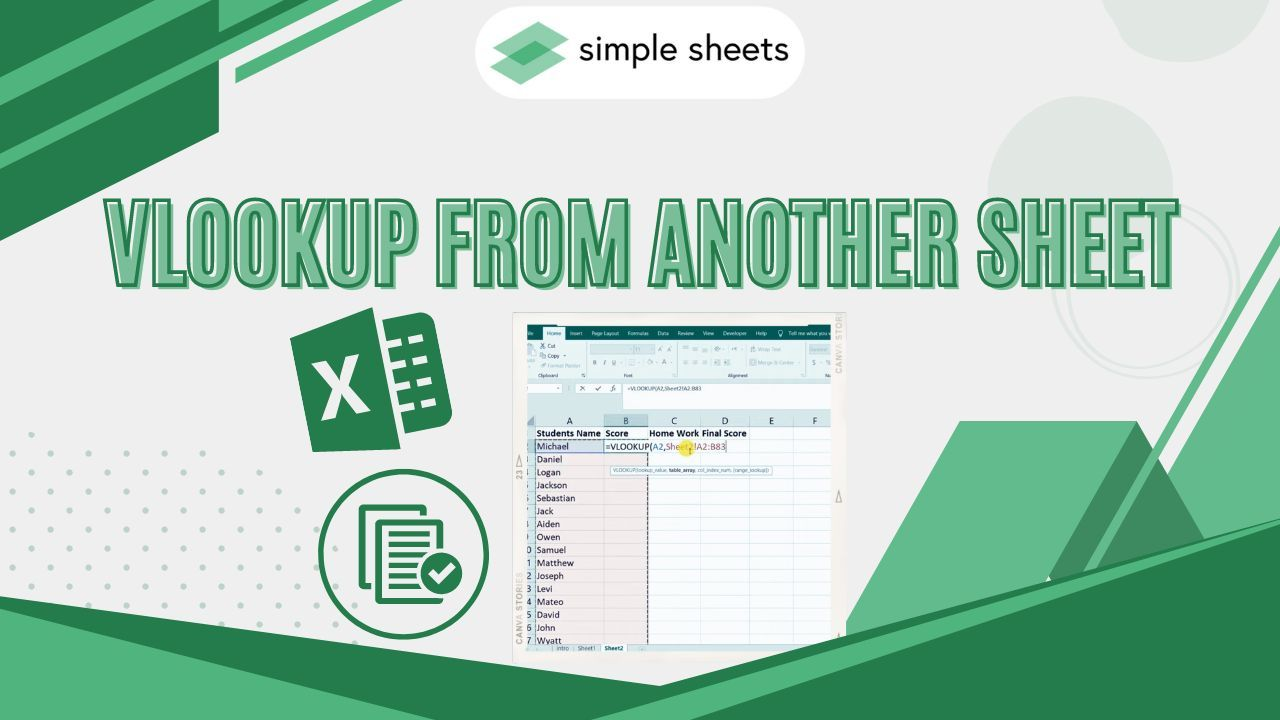Do you want to learn how to use VLOOKUP to retrieve data from a different sheet?

Well, you're in luck because, in this guide, we will show you how to do that. We'll teach you how to use VLOOKUP from another sheet while providing tips to maximize the features.

With our clear instructions and helpful examples, VLOOKUP will no longer be a challenging hurdle. Instead, it will become an invaluable tool for data analysis.

Read on as we cover the following:

• Excel VLOOKUP Function

• How to VLOOKUP from Another Sheet

• The Pros and Cons of Doing VLOOKUP from Another Sheet

• Important Details About the VLOOKUP Function

• Final Thoughts on Doing VLOOKUP from Another Sheet

• Frequently Asked Questions on VLOOKUP from Another Sheet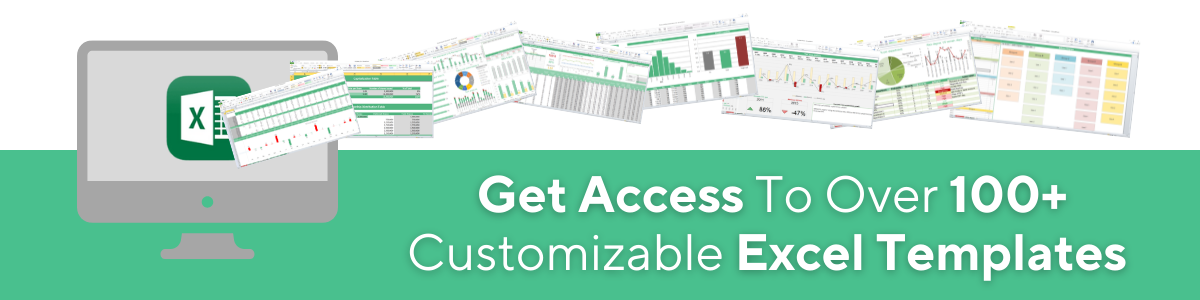## Excel VLOOKUP Function

The VLOOKUP function is widely used by Excel users working with multiple worksheets.

You can use it to return the data from another worksheet in an organized manner.

Moreover, VLOOKUP takes the lookup value and retrieves an exact match similar to the cell reference.

In general, VLOOKUP searches for a value in the first column of the chosen data range and retrieves the same value in the same row from the other column.

VLOOKUP is available from the 2007 to 2016 versions of Microsoft Excel.

### VLOOKUP function syntax

The image below represents the VLOOKUP function syntax: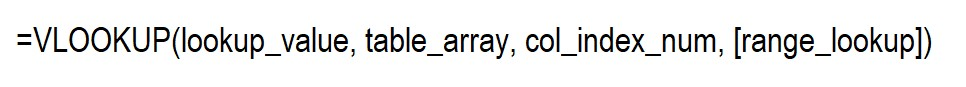• Lookup_value - you want to retrieve. A lookup value can be a value that has date, numerical, and text values, or it can also be a cell reference.

• Table_array - the selected range of cells to search data from or the table references. It can find case-sensitive values.

• Col_index_number - data must refer to the column within the table array range to search for the value.

• Range_lookup - the optional parameter, but it can be useful when retrieving matches. You can retrieve an approximate match with TRUE and choose FALSE to retrieve the exact match in the range lookup.

## How to VLOOKUP from Another Sheet

Consider the following example:

We have two worksheets in one data workbook. In Sheet 1, we have the players' names and jersey numbers, but the runs scored column is missing. We need to extract this column from Sheet 2.

Worksheet 1: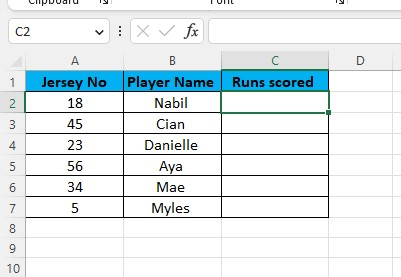Worksheet 2: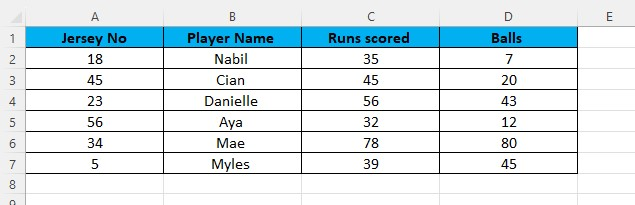As shown in the second worksheet sample, there are extra columns, such as Columns C and D.

So, importing data from Column C of Worksheet 2 to Worksheet 1 using VLOOKUP is necessary.

1. Type the VLOOKUP formula and the lookup value.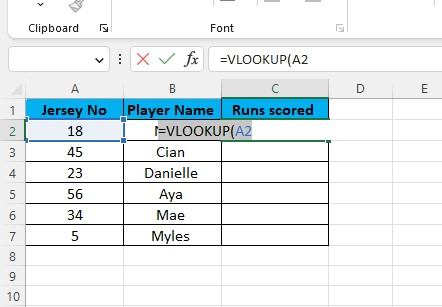1. Type the sheet name or the reference sheet.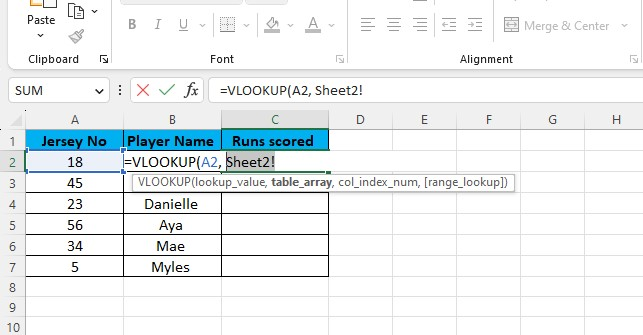1. Type or select the table range or the table array.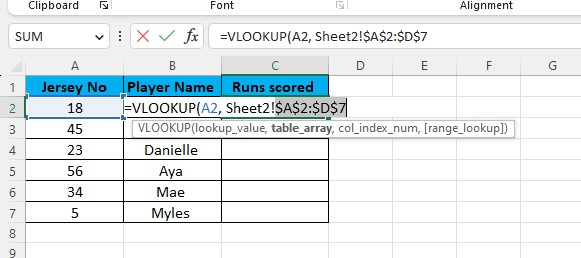1. Type the column number and the range lookup type, and input FALSE to retrieve the exact match.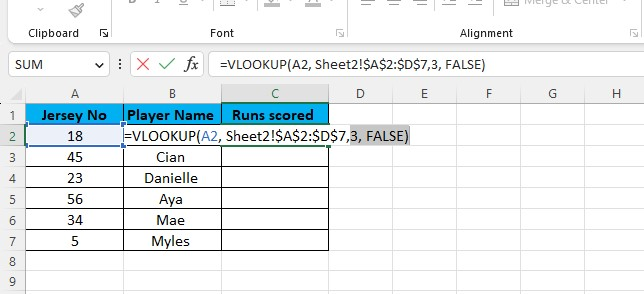1. Press the Enter key to retrieve data from the VLOOKUP formula.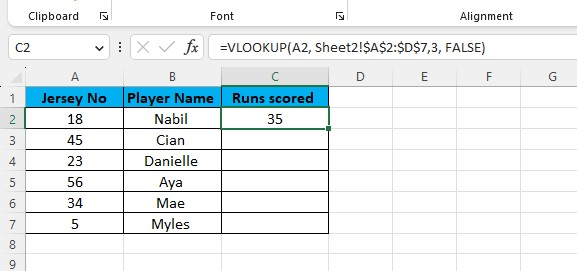1. Use the AutoFill command to fill out the other empty row.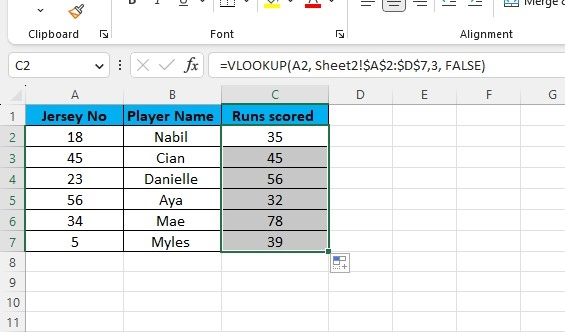Note: You can use VLOOKUP on the same worksheet.

## The Pros and Cons of Doing VLOOKUP from Another Sheet

The pros and cons of VLOOKUP from another datasheet are the following:

### Pros of VLOOKUP from another sheet

• VLOOKUP can assist the user in finding the value on the same sheet or from multiple sheets in the same workbook.

• VLOOKUP is better than the MATCH function because the MATCH function doesn't retrieve the value of the data but the cell reference.

• VLOOKUP can gather data from one worksheet to another.

• VLOOKUP can look at values from a different Excel file.

• VLOOKUP is not case-sensitive.

### Cons of VLOOKUP from another sheet

• Beginners may find VLOOKUP difficult to use because of its functions and parameters.

• If you need to use an approximate match, you have to sort the first column of table_array from low to high. It would be best if you sorted for it to give the right answer.

## Important Details About the VLOOKUP Function

These are the important details to remember about the VLOOKUP function:

• The VLOOKUP function does not search for data on the left side. Instead, it looks for the lookup value in the leftmost column and then continues its search from left to right.

• Remember that the formula will generate an error if we use a value less than 1 in the col_index_num parameter.

• To ensure formula accuracy when copying and pasting, use the '\$' symbol or the absolute cell references for the table_array parameter.

• You don't have to type the entire formula. Instead, you can switch to the sheet you need and use the mouse to select the range you need to use in the formula.

## Final Thoughts on Using VLOOKUP from Another Sheet

Using VLOOKUP across sheets greatly saves time and simplifies procedures. Moreover, it gives a simple and effective approach to collecting information from various workbooks.

Visit Simple Sheets for more easy-to-follow guides and examples, and remember to read the related articles section of this blog post.

For the most straightforward Excel video tutorials, subscribe to Simple Sheets on YouTube!## Frequently Asked Questions on VLOOKUP From Another Sheet

### What would happen if Excel doesn't recognize a VLOOKUP value?

If it doesn't recognize a VLOOKUP value in the first column of the table, VLOOKUP will retrieve an error value, such as #N/A.

### Are there any limitations or considerations when using VLOOKUP across sheets?

The column index number should be a positive integer corresponding to the column position in the table range.

### What would happen If I changed the table range of my VLOOKUP results?

If the data in the table range changes, the VLOOKUP result may also change. So, always ensure data integrity.## Related Articles

Excel Pivot Table Training: Everything You Need to Know

The Only Guide You'll Need For Excel Strikethrough

Excel is Fun

#### Want to Make Excel Work for You? Try out 5 Amazing Excel Templates & 5 Unique Lessons

We hate SPAM. We will never sell your information, for any reason.